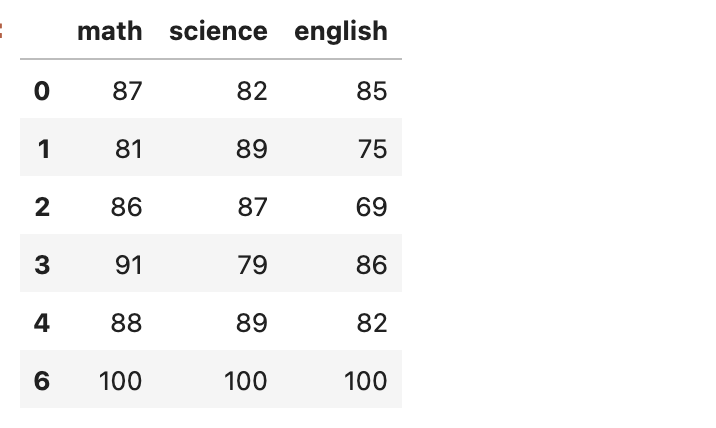# Python中寻找数据异常值的3种方法

## 2. 举个栗子

`data = pd.DataFrame([ [87, 82, 85], [81, 89, 75], [86, 87, 69], [91, 79, 86], [88, 89, 82], [0, 0, 0], # this guy missed the exam [100, 100, 100], ], columns=["math", "science", "english"])`## 3. 孤立森林

`from sklearn.ensemble import IsolationForest predictions = IsolationForest().fit(data).predict(data) # predictions = array([ 1, 1, 1, 1, 1, -1, -1])`

## 4. 椭圆模型拟合

`from sklearn.covariance import EllipticEnvelope predictions = EllipticEnvelope().fit(data).predict(data) # predictions = array([ 1, 1, 1, 1, 1, -1, 1])`

## 5. 局部异常因子算法

`from sklearn.neighbors import LocalOutlierFactor predictions = LocalOutlierFactor(n_neighbors=5, novelty=True).fit(data).predict(data) # array([ 1, 1, 1, 1, 1, -1, 1])`

## 7. 异常值消除

`# predictions = array([ 1, 1, 1, 1, 1, -1, 1]) data2 = data[predictions==1]`## 相关推荐

• 简要pyinstaller模块主要用于python代码打包成exe程序直接使用，这样在其它电脑上即使没有python环
04月25日 10:22

HOT